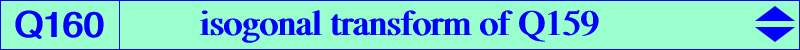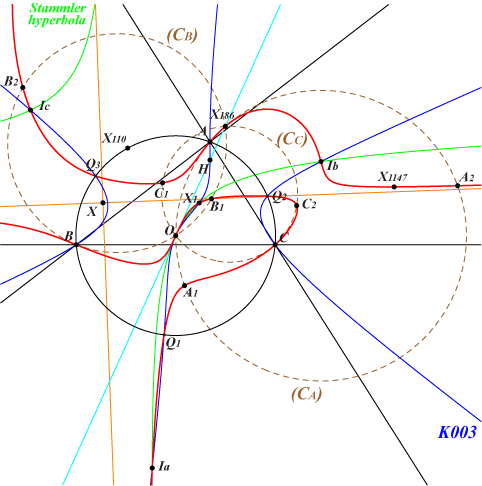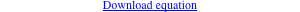too complicated to be written here. Click on the link to download a text file.X(1), X(3), X(186), X(1147), X(2574), X(2575) excenters circular points at infinity J1, J2 Q1, Q2, Q3 : vertices of the CircumNormal triangle, see Table 25 isogonal conjugates of the Ix-anticevian points, see Table 23 other points belowThe isogonal transform of the circular quintic Q159 is Q160. Q160 is a circular circum-quartic with two imaginary asymptotes passing through X(186) and two real asymptotes parallel to those of the Jerabek hyperbola. These meet at X on the lines {X5, X578}, {X30, X110}, {X49, X140}, {X155, X1192}, {X156, X550}, etc, SEARCH = 2.54688454278036. Q160 is a member of the pencil of circular circum-quartics generated by the two following decomposed curves : union of K003 and the line at infinity, union of the circum-circle and the Stammler diagonal hyperbola. The circle (CA) passing through A, X(3), X(186) is tangent at A to Q160. It meets the A-bisectors of ABC at A1, A2 on Q160. The points B1, B2 and C1, C2 are defined similarly. Q160 meets the Jerabek hyperbola at A, B, C, O, X(2574), X(2575) and two other points on the line{578, 1199, 1204, etc}. *** Q160 and the Euler pencil, see Table 27 Every cubic (K) of the Euler pencil is a pK(X6, P) with P on the Euler line. It meets Q160 at 8 fixed points namely A, B, C, X(1), X(3) and the excenters. Hence, it must have 4 other common points with Q160. The following table gives a few remarkable examples.(K) the four points K001 J1, J2 and N1, N2 on the line {3, 1147), on the circum-conic through {254, 1138} K003 Q1, Q2, Q3 and O again since Q160 is tangent at O to the Euler line K005 isogonal conjugates of the Ix-anticevian points